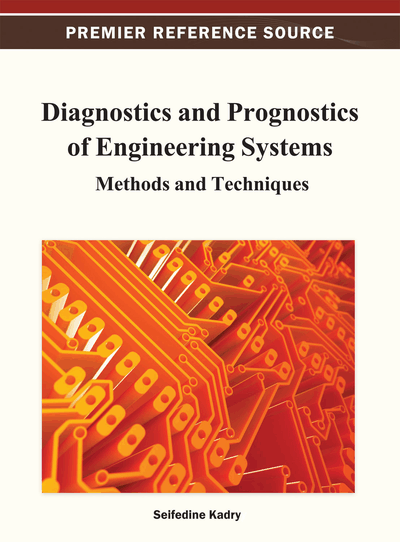# Iterative Fault Tolerant Control for General Discrete-Time Stochastic Systems Using Output Probability Density Estimation

Zakwan Skaf (University of Manchester, UK)
DOI: 10.4018/978-1-4666-2095-7.ch001
Available
\$37.50
No Current Special Offers

## Abstract

A new design of a fault tolerant control (FTC)-based an adaptive, fixed-structure proportional-integral (PI) controller with constraints on the state vector for nonlinear discrete-time system subject to stochastic non-Gaussian disturbance is studied. The objective of the reliable control algorithm scheme is to design a control signal such that the actual probability density function (PDF) of the system is made as close as possible to a desired PDF, and make the tracking performance converge to zero, not only when all components are functional but also in case of admissible faults. A Linear Matrix Inequality (LMI)-based FTC method is presented to ensure that the fault can be estimated and compensated for. A radial basis function (RBF) neural network is used to approximate the output PDF of the system. Thus, the aim of the output PDF control will be a RBF weight control with an adaptive tuning of the basis function parameters. The key issue here is to divide the control horizon into a number of equal time intervals called batches. Within each interval, there are a fixed number of sample points. The design procedure is divided into two main algorithms, within each batch, and between any two adjacent batches. A P-type Iterative Learning Control (ILC) law is employed to tune the parameters of the RBF neural network so that the PDF tracking error decreases along with the batches. Sufficient conditions for the proposed fault tolerance are expressed as LMIs. An analysis of the ILC convergence is carried out. Finally, the effectiveness of the proposed method is demonstrated with an illustrated example.
Chapter Preview
Top

## 1. Introduction

Under the assumption that the random variables or the noise in the stochastic system are subject to Gaussian processes, the following approaches have been widely applied in theoretical studies: minimum variance control (Astrom, 1970), whose purpose is minimizing the variations in the controlled system outputs or tracking errors, linear optimal control (Anderson, 1971), linear quadratic martingale control (Solo, 1990), and stochastic control for systems with Markovian jump parameters (Xia, Shi, Liu, and Rees, 2006). In all these methods, the targets are the mean and variance of the output. However, this assumption may not hold in some applications. For example, many variables in the paper-making systems do not obey Gaussian distributions (Wang, 2000, Wang,1999). Therefore, a new measure of randomness, called the PDF control, should be employed for general stochastic systems with non-Gaussian variables (Wang, 2000). In PDF control problems, the control objective is to design a control signal so that the PDF shape of the output variable follows a desired distribution.

There are many stochastic systems in practice whose outputs are the PDF of the system output (Wang, 2000) rather than the actual output values. For such cases, the measured output PDFs can be used as an output for the feedback control. Such types of stochastic systems are called Stochastic Distribution Control (SDC) systems (Wang, 2000). Practical examples of SDC systems in industrial applications include: Molecular weight distribution control (Crowley and Choi, 1998, Shibasaki, Araki, Nagahata, and Ueda, 2005), combustion flame distribution processes (Sun, Yue, and Wang, 2006, Wang, Afshar, and Wang, 2008), particle size distribution control in polymerisation and powder processing industries (Dunbar and Hickey, 2000, Shi, El-Farra, Li, Mhaskar, and Christo□des, 2006), and the wet-end of paper-making (Wang, 2000). A well-known example of a SDC system in practice is the 2D paper web solid distribution in paper-making processes (Wang, 2000). For instance, the PDF distribution of the grammage of the finished sheet can be measured online via digital cameras. Such images from these digital cameras can be processed and used as a feedback signal to the control system (Wang, 2000).

SDC was originally developed by Professor Hong Wang in 1996, when he considered a number of challenging paper machine modelling and control problems (Wang, 2000). The process and the control were presented in a PDF form. As such, the purpose of the controller design was to obtain the PDF of the controller so that the closed-loop PDF would follow the pre-specified PDF. Since then, rapid developments have been made and introduced in different control applications (A. Wang, H. Wang, and Guo, 2009).

The most existing PDF control approaches are based on the B-spline model. Moreover, multi-layer perception (MLP) neural network models have been applied to the shape control for the output PDFs (Wang, 2003). Recently, a RBF neural network has been used to approximate the output PDF of the system (Wang and Afshar, 2009). In this work, we have used RBFs instead of B-Splines which help generalize the output PDF expression and overcome the complexity, limitations, and constrains with B-spline-based functional approximations.

## Complete Chapter List

Search this Book:
Reset